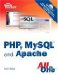# Workshop

The workshop is designed to help you anticipate possible questions, review what you've learned, and begin putting your knowledge into practice.

#### Quiz

 1 True or false: If a function doesn't require an argument, you can omit the parentheses in the function call. 2 How do you return a value from a function? 3 What would the following code fragment print to the browser? `\$number = 50; function tenTimes() { \$number = \$number * 10; } tenTimes(); echo \$number;` 4 What would the following code fragment print to the browser? `\$number = 50; function tenTimes() { global \$number; \$number = \$number * 10; } tenTimes(); echo \$number;` 5 What would the following code fragment print to the browser? `\$number = 50; function tenTimes( &\$n ) { \$n = \$n * 10; } tenTimes( \$number ); echo \$number;`

 1 The statement is false. You must always include the parentheses in your function calls, whether you are passing arguments to the function or not. 2 You must use the return keyword. 3 It would print 50. The tenTimes() function has no access to the global \$number variable. When it is called, it will manipulate its own local \$number variable. 4 It would print 500. We have used the global statement, which gives the tenTimes() function access to the \$number variable. 5 It would print 500. By adding the ampersand to the parameter variable \$n, we ensure that this argument is passed by reference. \$n and \$number point to the same value, so any changes to \$n will be reflected when you access \$number.

#### Activity

Create a function that accepts four string variables and returns a string that contains an HTML table element, enclosing each of the variables in its own cell.Sams Teach Yourself PHP, MySQL and Apache All in One (3rd Edition)
ISBN: 0672328739
EAN: 2147483647
Year: 2004
Pages: 327